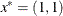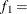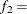### Example 7.4 Restarting an Optimization

This example shows how you can restart an optimization problem using the OUTEST=, INEST=, OUTMODEL=, and MODEL= options and how to save output into an OUT= data set. The least squares solution of the Rosenbrock function using the trust region method is used.

The following code solves the problem and saves the model in the MODEL data set and the solution in the EST and OUT1 data sets.

```
proc nlp tech=trureg outmodel=model outest=est out=out1;
lsq y1 y2;
parms x1 = -1.2 ,
x2 =  1.;
y1 = 10. * (x2 - x1 * x1);
y2 = 1. - x1;
run;
```
```proc print data=out1;
run;
```

The final parameter estimatesand the values of the functionsY1 andY2 are written into an OUT= data set, shown in Output 7.4.1. Since OUTDER=0 is the default, the OUT= data set does not contain the Jacobian matrix.

Output 7.4.1: Solution in an OUT= Data Set

Obs _OBS_ _TYPE_ y1 y2 x2 x1
1 1   0 -2.2204E-16 1 1

Next, the procedure reads the optimal parameter estimates from the EST data set and the model from the MODEL data set. It does not do any optimization (TECH=NONE), but it saves the Jacobian matrix to the OUT=OUT2 data set because of the option OUTDER=1. It also displays the Jacobian matrix because of the option PJAC; the Jacobian matrix is shown in Output 7.4.2. Output 7.4.3 shows the contents of the OUT2 data set, which also contains the Jacobian matrix.

```proc nlp tech=none model=model inest=est out=out2 outder=1 pjac PHISTORY;
lsq y1 y2;
parms x1 x2;
run;
```
```proc print data=out2;
run;
```

Output 7.4.2: Jacobian Matrix Output

PROC NLP: Least Squares Minimization

Jacobian Matrix
x1 x2
-20 10
-1 0

Output 7.4.3: Jacobian Matrix in an OUT= Data Set

Obs _OBS_ _TYPE_ y1 y2 _WRT_ x2 x1
1 1   0 -0   1 1
2 1 ANALYTIC 10 0 x2 1 1
3 1 ANALYTIC -20 -1 x1 1 1